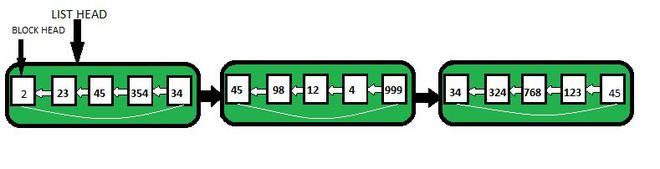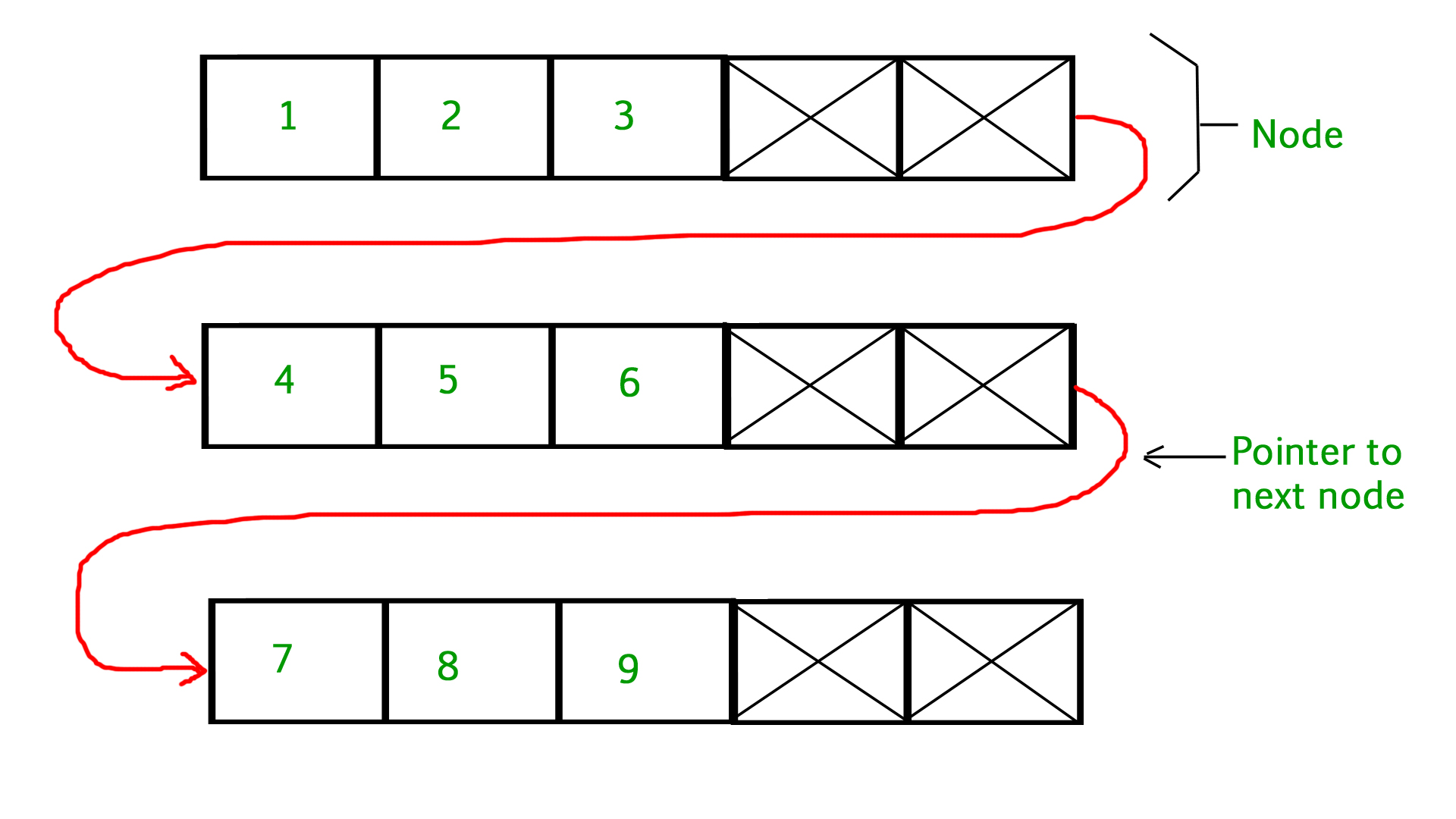Open In App
Related Articles

# Unrolled Linked List | Set 1 (Introduction)

Like array and linked list, the unrolled Linked List is also a linear data structure and is a variant of a linked list.

Why do we need unrolled linked list?• Because of the Cache behavior, linear search is much faster in unrolled linked lists.
• In comparison to the ordinary linked list, it requires less storage space for pointers/references.
• It performs operations like insertion, deletion, and traversal more quickly than ordinary linked lists (because search is faster).

• The overhead per node is comparatively high than singly-linked lists. Refer to an example node in the below code

Example: Let say we are having 8 elements so sqrt(8)=2.82 which rounds off to 3. So each block will store 3 elements. Hence, to store 8 elements 3 blocks will be created out of which the first two blocks will store 3 elements and the last block would store 2 elements.

How searching becomes better in unrolled linked lists?

So taking the above example if we want to search for the 7th element in the list we traverse the list of blocks to the one that contains the 7th element. It takes only O(sqrt(n)) since we found it through not going more than sqrt(n) blocks.

Simple Implementation:

The below program creates a simple unrolled linked list with 3 nodes containing a variable number of elements in each. It also traverses the created list.

## C++

 `// C++ program to implement unrolled linked list ``// and traversing it. ``#include ``using` `namespace` `std;``#define maxElements 4 `` ` `// Unrolled Linked List Node ``class` `Node ``{ ``    ``public``:``    ``int` `numElements; ``    ``int` `array[maxElements]; ``    ``Node *next; ``}; `` ` `/* Function to traverse an unrolled linked list ``and print all the elements*/``void` `printUnrolledList(Node *n) ``{ ``    ``while` `(n != NULL) ``    ``{ ``        ``// Print elements in current node ``        ``for` `(``int` `i=0; inumElements; i++) ``            ``cout<array[i]<<``" "``; `` ` `        ``// Move to next node ``        ``n = n->next; ``    ``} ``} `` ` `// Program to create an unrolled linked list ``// with 3 Nodes ``int` `main() ``{ ``    ``Node* head = NULL; ``    ``Node* second = NULL; ``    ``Node* third = NULL; `` ` `    ``// allocate 3 Nodes ``    ``head = ``new` `Node();``    ``second = ``new` `Node();``    ``third = ``new` `Node();`` ` `    ``// Let us put some values in second node (Number ``    ``// of values must be less than or equal to ``    ``// maxElement) ``    ``head->numElements = 3; ``    ``head->array = 1; ``    ``head->array = 2; ``    ``head->array = 3; `` ` `    ``// Link first Node with the second Node ``    ``head->next = second; `` ` `    ``// Let us put some values in second node (Number ``    ``// of values must be less than or equal to ``    ``// maxElement) ``    ``second->numElements = 3; ``    ``second->array = 4; ``    ``second->array = 5; ``    ``second->array = 6; `` ` `    ``// Link second Node with the third Node ``    ``second->next = third; `` ` `    ``// Let us put some values in third node (Number ``    ``// of values must be less than or equal to ``    ``// maxElement) ``    ``third->numElements = 3; ``    ``third->array = 7; ``    ``third->array = 8; ``    ``third->array = 9; ``    ``third->next = NULL; `` ` `    ``printUnrolledList(head); `` ` `    ``return` `0; ``} `` ` `// This is code is contributed by rathbhupendra`

## C

 `// C program to implement unrolled linked list``// and traversing it.``#include``#include``#define maxElements 4`` ` `// Unrolled Linked List Node``struct` `Node``{``    ``int` `numElements;``    ``int` `array[maxElements];``    ``struct` `Node *next;``};`` ` `/* Function to traverse an unrolled linked list``   ``and print all the elements*/``void` `printUnrolledList(``struct` `Node *n)``{``    ``while` `(n != NULL)``    ``{``        ``// Print elements in current node``        ``for` `(``int` `i=0; inumElements; i++)``            ``printf``(``"%d "``, n->array[i]);`` ` `        ``// Move to next node ``        ``n = n->next;``    ``}``}`` ` `// Program to create an unrolled linked list``// with 3 Nodes``int` `main()``{``    ``struct` `Node* head = NULL;``    ``struct` `Node* second = NULL;``    ``struct` `Node* third = NULL;`` ` `    ``// allocate 3 Nodes``    ``head = (``struct` `Node*)``malloc``(``sizeof``(``struct` `Node));``    ``second = (``struct` `Node*)``malloc``(``sizeof``(``struct` `Node));``    ``third = (``struct` `Node*)``malloc``(``sizeof``(``struct` `Node));`` ` `    ``// Let us put some values in second node (Number``    ``// of values must be less than or equal to``    ``// maxElement)``    ``head->numElements = 3;``    ``head->array = 1;``    ``head->array = 2;``    ``head->array = 3;`` ` `    ``// Link first Node with the second Node``    ``head->next = second;`` ` `    ``// Let us put some values in second node (Number``    ``// of values must be less than or equal to``    ``// maxElement)``    ``second->numElements = 3;``    ``second->array = 4;``    ``second->array = 5;``    ``second->array = 6;`` ` `    ``// Link second Node with the third Node``    ``second->next = third;`` ` `    ``// Let us put some values in third node (Number``    ``// of values must be less than or equal to``    ``// maxElement)``    ``third->numElements = 3;``    ``third->array = 7;``    ``third->array = 8;``    ``third->array = 9;``    ``third->next = NULL;`` ` `    ``printUnrolledList(head);`` ` `    ``return` `0;``}`

## Java

 `// Java program to implement unrolled``// linked list and traversing it. ``import` `java.util.*;`` ` `class` `GFG{``     ` `static` `final` `int` `maxElements = ``4``;`` ` `// Unrolled Linked List Node ``static` `class` `Node ``{ ``    ``int` `numElements; ``    ``int` `[]array = ``new` `int``[maxElements]; ``    ``Node next; ``}; `` ` `// Function to traverse an unrolled ``// linked list  and print all the elements``static` `void` `printUnrolledList(Node n) ``{ ``    ``while` `(n != ``null``) ``    ``{ ``       ` `        ``// Print elements in current node ``        ``for``(``int` `i = ``0``; i < n.numElements; i++) ``            ``System.out.print(n.array[i] + ``" "``); `` ` `        ``// Move to next node ``        ``n = n.next; ``    ``} ``} `` ` `// Program to create an unrolled linked list ``// with 3 Nodes ``public` `static` `void` `main(String[] args) ``{ ``    ``Node head = ``null``; ``    ``Node second = ``null``; ``    ``Node third = ``null``; `` ` `    ``// Allocate 3 Nodes ``    ``head = ``new` `Node();``    ``second = ``new` `Node();``    ``third = ``new` `Node();`` ` `    ``// Let us put some values in second ``    ``// node (Number of values must be ``    ``// less than or equal to maxElement) ``    ``head.numElements = ``3``; ``    ``head.array[``0``] = ``1``; ``    ``head.array[``1``] = ``2``; ``    ``head.array[``2``] = ``3``; `` ` `    ``// Link first Node with the ``    ``// second Node ``    ``head.next = second; `` ` `    ``// Let us put some values in ``    ``// second node (Number of values``    ``// must be less than or equal to ``    ``// maxElement) ``    ``second.numElements = ``3``; ``    ``second.array[``0``] = ``4``; ``    ``second.array[``1``] = ``5``; ``    ``second.array[``2``] = ``6``; `` ` `    ``// Link second Node with the third Node ``    ``second.next = third; `` ` `    ``// Let us put some values in third ``    ``// node (Number of values must be``    ``// less than or equal to maxElement) ``    ``third.numElements = ``3``; ``    ``third.array[``0``] = ``7``; ``    ``third.array[``1``] = ``8``; ``    ``third.array[``2``] = ``9``; ``    ``third.next = ``null``; `` ` `    ``printUnrolledList(head); ``} ``} `` ` `// This code is contributed by amal kumar choubey  `

## Python3

 `# Python3 program to implement unrolled``# linked list and traversing it. ``maxElements ``=` `4` ` ` `# Unrolled Linked List Node ``class` `Node:``     ` `    ``def` `__init__(``self``):``         ` `        ``self``.numElements ``=` `0``        ``self``.array ``=` `[``0` `for` `i ``in` `range``(maxElements)] ``        ``self``.``next` `=` `None`` ` `# Function to traverse an unrolled linked list ``# and print all the elements``def` `printUnrolledList(n):`` ` `    ``while` `(n !``=` `None``):`` ` `        ``# Print elements in current node ``        ``for` `i ``in` `range``(n.numElements):``            ``print``(n.array[i], end ``=` `' '``)`` ` `        ``# Move to next node ``        ``n ``=` `n.``next`` ` `# Driver Code``if` `__name__``=``=``'__main__'``:``     ` `    ``head ``=` `None``    ``second ``=` `None` `    ``third ``=` `None` ` ` `    ``# Allocate 3 Nodes ``    ``head ``=` `Node()``    ``second ``=` `Node()``    ``third ``=` `Node()`` ` `    ``# Let us put some values in second``    ``# node (Number of values must be ``    ``# less than or equal to ``    ``# maxElement) ``    ``head.numElements ``=` `3``    ``head.array[``0``] ``=` `1``    ``head.array[``1``] ``=` `2` `    ``head.array[``2``] ``=` `3` ` ` `    ``# Link first Node with the second Node ``    ``head.``next` `=` `second`` ` `    ``# Let us put some values in second node``    ``# (Number of values must be less than``    ``# or equal to maxElement) ``    ``second.numElements ``=` `3``    ``second.array[``0``] ``=` `4``    ``second.array[``1``] ``=` `5` `    ``second.array[``2``] ``=` `6` ` ` `    ``# Link second Node with the third Node ``    ``second.``next` `=` `third `` ` `    ``# Let us put some values in third node``    ``# (Number of values must be less than ``    ``# or equal to maxElement) ``    ``third.numElements ``=` `3``    ``third.array[``0``] ``=` `7``    ``third.array[``1``] ``=` `8` `    ``third.array[``2``] ``=` `9` `    ``third.``next` `=` `None` ` ` `    ``printUnrolledList(head)``     ` `# This code is contributed by rutvik_56`

## C#

 `// C# program to implement unrolled``// linked list and traversing it. ``using` `System;``class` `GFG{``     ` `static` `readonly` `int` `maxElements = 4;`` ` `// Unrolled Linked List Node ``class` `Node ``{ ``  ``public` `int` `numElements; ``  ``public` `int` `[]array = ``new` `int``[maxElements]; ``  ``public` `Node next; ``}; `` ` `// Function to traverse an unrolled ``// linked list  and print all the elements``static` `void` `printUnrolledList(Node n) ``{ ``  ``while` `(n != ``null``) ``  ``{ ``    ``// Print elements in current node ``    ``for``(``int` `i = 0; i < n.numElements; i++) ``      ``Console.Write(n.array[i] + ``" "``); `` ` `    ``// Move to next node ``    ``n = n.next; ``  ``} ``} `` ` `// Program to create an unrolled linked list ``// with 3 Nodes ``public` `static` `void` `Main(String[] args) ``{ ``  ``Node head = ``null``; ``  ``Node second = ``null``; ``  ``Node third = ``null``; `` ` `  ``// Allocate 3 Nodes ``  ``head = ``new` `Node();``  ``second = ``new` `Node();``  ``third = ``new` `Node();`` ` `  ``// Let us put some values in second ``  ``// node (Number of values must be ``  ``// less than or equal to maxElement) ``  ``head.numElements = 3; ``  ``head.array = 1; ``  ``head.array = 2; ``  ``head.array = 3; `` ` `  ``// Link first Node with the ``  ``// second Node ``  ``head.next = second; `` ` `  ``// Let us put some values in ``  ``// second node (Number of values``  ``// must be less than or equal to ``  ``// maxElement) ``  ``second.numElements = 3; ``  ``second.array = 4; ``  ``second.array = 5; ``  ``second.array = 6; `` ` `  ``// Link second Node with the third Node ``  ``second.next = third; `` ` `  ``// Let us put some values in third ``  ``// node (Number of values must be``  ``// less than or equal to maxElement) ``  ``third.numElements = 3; ``  ``third.array = 7; ``  ``third.array = 8; ``  ``third.array = 9; ``  ``third.next = ``null``; `` ` `  ``printUnrolledList(head); ``} ``} `` ` `// This code is contributed by Rajput-Ji`

## Javascript

 ``

Output

`1 2 3 4 5 6 7 8 9 `

Complexity Analysis:

• Time Complexity: O(n).
• Space Complexity: O(n).

In this article, we have introduced an unrolled list and advantages of it. We have also shown how to traverse the list. In the next article, we will be discussing the insertion, deletion, and values of maxElements/numElements in detail.